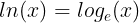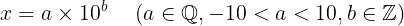# ln(x): calculation and implementation in different programming languages

The natural logarithm of a number is that number’s logarithm to the base of e (a mathematical constant). Shown as a mathematical statement:The natural logarithm of a number is that number’s logarithm to the base of e

To show this better, we could also use:

Now, we go back to our first problem: “How to calculate ln(x)?”

For the first step, we are going to find the Maclaurin’s series in order to find our Answer:

So if -1≤t≤1:

And for the final step:

We can also show this equation by:

In order to calculate ln(x) itself, we use a simple trick: setting the value of (x) to (t-1):

As conclusion:

But if you remember, we said that this equation only works if -1≤x≤1.

So how can we rewrite this formula to calculate ln(x), for x ∈ ℝ?

But first, let’s look into some basic rules of natural logarithm:

Now back to our own problem, we can show each number as a scientific notation. Shown as a mathematical expression:we can show each number as a scientific notation, ‘a’ is called “Coefficient” and ‘b’ is called “Exponent”.

For example:

Now, let’s modify this scientific notation:

Since -10<a<10, then -1<0.1a<1. As said before, our ln(x) equation worked only if -1≤x≤1. Then:

since 10 isn’t less than 1:

One quick look at what we got:

Now let’s calculate each part with the expression we had before:

Concluding:

Now that we have the final equation, let’s get into implementing it in Node.js and Python 3.8.

# Implementation in Python 3.8

Implementation of the ln function in Python is shown below. First we convert x to its scientific notation, then we do the rest of calculations. Note that since we can’t calculate this to infinity, we have to end our calculations at some point; So we set our limit to endOfCalc. Obviously, the larger endOfCalc is, the more accurate our result will be. For example, I set endOfCalc to 370 because it still runs smoothly and gives us the result with the accuracy of 10 digits after the decimal point (at most). Take a look:

`endOfCalc = 370def ln(x):    scientific_notation = "{:.12e}".format(x)    scientific_notation = scientific_notation.replace('+','')    a, b = map(float, scientific_notation.split('e'))    output = 0    for i in range(1,endOfCalc):        output += (((a/10)-1)**i)*((-1)**(i+1))/i        output -= (b+1)*((-0.9)**i)*((-1)**(i+1))/i    return round(output,10)`

Now to test it, we add ln(e) (e is approximately equal to 2.718281828459) to the program:

`print(ln(2.718281828459))`

When you run it, you’ll get 1.0; Means that everything worked out just fine.

A wise thing to do in order to reduce the execution time, is to pre-calculate ln(0.1), which is approximately equal to -2.30258509299. So the final code is:

`endOfCalc = 370ln0_1 = -2.30258509299;def ln(x):    scientific_notation = "{:.12e}".format(x)    scientific_notation = scientific_notation.replace('+','')    a, b = map(float, scientific_notation.split('e'))    output = 0    for i in range(1,endOfCalc):        output += (((a/10)-1)**i)*((-1)**(i+1))/i    return round(output-(b+1)*ln0_1,10)`

Again, you can test it with the method explained before.

# Implementation in Node.js

Implementation of the ln function in Node.js is shown below. First we convert x to its scientific notation, then we do the rest of calculations. Note that since we can’t calculate this to infinity, we have to end our calculations at some point; So we set our limit to endOfCalc. Obviously, the larger endOfCalc is, the more accurate our result will be. For example, I set endOfCalc to 370 because it still runs smoothly and gives us the result with the accuracy of 10 digits after the decimal point. Take a look:

`endOfCalc = 370;function ln(x){    x = x.toExponential();    x = x .replace('+','');    let listed = x.split("e");    a = Number(listed);b = Number(listed);    output = 0;    for(i = 1;i < endOfCalc;i++){        output += (((a/10)-1)**i)*((-1)**(i+1))/i;        output -= (b+1)*((-0.9)**i)*((-1)**(i+1))/i;    }    return (output).toFixed((10));}`

Now to test it, we add ln(e) (e is approximately equal to 2.718281828459) to the program:

`console.log(ln(2.718281828459))`

When you run it, you’ll get 1.0000000000; Means that everything worked out just fine.

A wise thing to do in order to reduce the execution time, is to pre-calculate ln(0.1), which is approximately equal to -2.30258509299. So the final code is:

`endOfCalc = 370;ln0_1 = -2.30258509299;function ln(x){    x = x.toExponential();    x = x .replace('+','');    let listed = x.split("e");    a = Number(listed);b = Number(listed);    output = 0;    for(i = 1;i < endOfCalc;i++){        output+=(((a/10)-1)**i)*((-1)**(i+1))/i;    }    return (output-(b+1)*ln0_1).toFixed((10));}`

Again, you can test it with the method explained before.

Shahid Ezheie 1 Highschool student, Iran

## More from Mani Ebrahimi

Shahid Ezheie 1 Highschool student, Iran